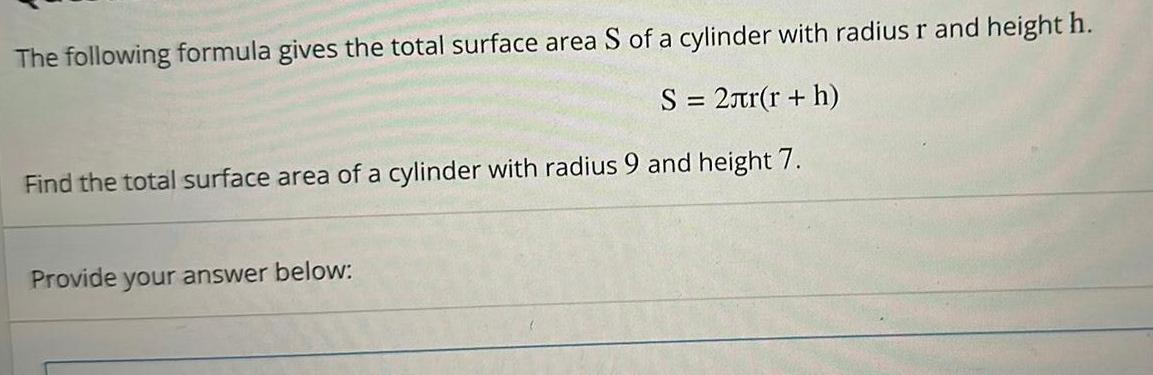Question:

# The following formula gives the total surface area S of a

Last updated: 5/26/2023The following formula gives the total surface area S of a cylinder with radius r and height h S 2 r r h Find the total surface area of a cylinder with radius 9 and height 7 Provide your answer below